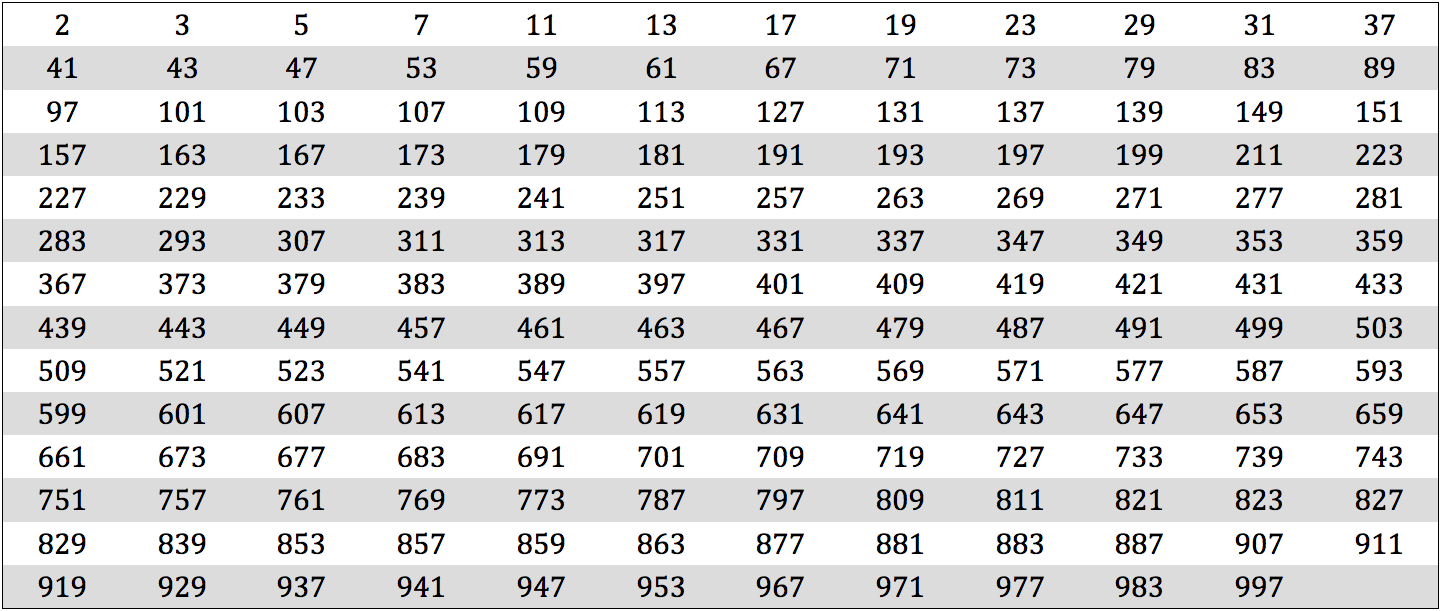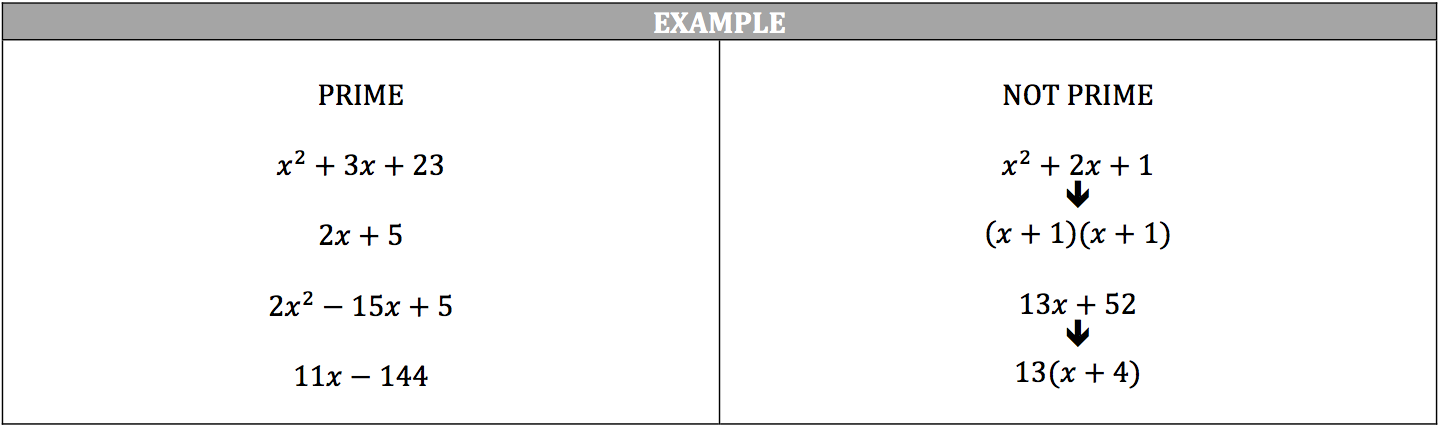A prime number is a positive number that has only two factors: 1 and the number itself. Zero is not prime because it has many factors (any number times zero is zero). One is not prime because it only has one factor: the number 1. The only even prime number is 2 since any even number can be divided by 2. Remember, prime factors are prime numbers that are the factors of another number, such as 5 and 7 are prime factors of 35.

Think about the following to determine if a number is prime or not:

• If the one’s place digit is even, then the number is not a prime number (it is a multiple of 2)
• If the one’s place digit is a 5, then the number is not a prime number (it is a multiple of 5)
• If the sum of the number’s digits is a multiple of 3 (153 → 1 + 5 + 3 = 9), then the number is not a prime number (it is a multiple of 3)
• Try to divide the number by some of the basic prime numbers, such as 2, 3, 5, 7, and 11. If the number is divisible by any of these numbers, then the number is not a prime number

Review this table of prime numbers between 0 and 1000:The concept of prime numbers also applies to algebraic expressions. If an algebraic expression cannot be factored out then it is considered prime.